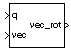# Quaternion Rotation

Rotate vector by quaternion

• Library:
• Aerospace Blockset / Utilities / Math Operations

•## Description

The Quaternion Rotation block rotates a vector by a quaternion. Aerospace Blockset™ uses quaternions that are defined using the scalar-first convention. This block normalizes all quaternion inputs. For the equations used for the quaternion, vector, and rotated vector, see Algorithms.

## Ports

### Input

expand all

Quaternions in the form of [q0, r0, ..., q1, r1, ... , q2, r2, ... , q3, r3, ...], specified as a quaternion or vector of quaternions.

Data Types: `double`

Vector or vector of vectors in the form of [v1, u1, ... , v2, u2, ... , v3, u3, ...].

Data Types: `double`

### Output

expand all

Rotated vector or vector of rotated vectors.

Data Types: `double`

## Algorithms

The quaternion has the form of

`$q={q}_{0}+i{q}_{1}+j{q}_{2}+k{q}_{3}.$`

The vector has the form of

`$v=i{v}_{1}+j{v}_{2}+k{v}_{3}.$`

The rotated vector has the form of

`${v}^{\prime }=\left[\begin{array}{c}{v}_{1}{}^{\prime }\\ {v}_{2}{}^{\prime }\\ {v}_{3}{}^{\prime }\end{array}\right]=\left[\begin{array}{ccc}\left(1-2{q}_{2}^{2}-2{q}_{3}^{2}\right)& 2\left({q}_{1}{q}_{2}+{q}_{0}{q}_{3}\right)& 2\left({q}_{1}{q}_{3}-{q}_{0}{q}_{2}\right)\\ 2\left({q}_{1}{q}_{2}-{q}_{0}{q}_{3}\right)& \left(1-2{q}_{1}^{2}-2{q}_{3}^{2}\right)& 2\left({q}_{2}{q}_{3}+{q}_{0}{q}_{1}\right)\\ 2\left({q}_{1}{q}_{3}+{q}_{0}{q}_{2}\right)& 2\left({q}_{2}{q}_{3}-{q}_{0}{q}_{1}\right)& \left(1-2{q}_{1}^{2}-2{q}_{2}^{2}\right)\end{array}\right]\left[\begin{array}{c}{v}_{1}\\ {v}_{2}\\ {v}_{3}\end{array}\right]$`

 Stevens, Brian L., Frank L. Lewis. Aircraft Control and Simulation, Second Edition. Hoboken, NJ: Wiley–Interscience.

 Diebel, James. "Representing Attitude: Euler Angles, Unit Quaternions, and Rotation Vectors." Stanford University, Stanford, California, 2006.